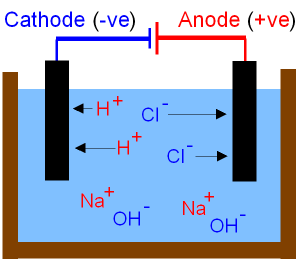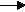gcsescience.com                                       11                                       gcsescience.com

The Periodic Table

The Alkali Metals - Electrolysis of Sodium Chloride in Water.

which has been dissolved in water. You get
different products if the s
odium chloride is completely dry.

What is the Electrolysis of Brine?

Sodium chloride dissolved in water is called brine.
Electrolysis of brine gives hydrogen gas at the cathode and
chlorine gas at the anode. You must know how to test
for hydrogen and chlorine gas. Sodium hydroxide remains
dissolved in the solution. The picture below shows
what happens to the ions during the electrolysis of brine.
This is an important industrial process making
hydrogen gas, chlorine gas and sodium hydroxide.What are Half Equations?

The reactions at each electrode are called half equations.
The half equations are written so that
the same number of electrons occur in each equation.

2H+  +  2e-H2 (hydrogen gas at the (-)cathode).
2
Cl-  -  2e-Cl2  (chlorine gas at the (+)anode).

Hydrogen ions gain electrons (reduction) to form hydrogen atoms.
The hydrogen atoms combine to form molecules of hydrogen gas.

Chloride ions lose electrons (oxidation) to form chlorine atoms.
The chlorine atoms combine to form molecules of chlorine gas.

The overall reaction is
2NaCl(aq)  +  2H2O(l)2Na+(aq) +  2OH-(aq) +  Cl2(g) +  H2(g)

See more detail of the electrolysis on the next page.

The electrolysis of hydrochloric acid also gives
hydrogen at the cathode and chlorine at the anode but
hydrochloric acid is more expensive than brine
and you do not get sodium hydroxide left in the solution.

gcsescience.com    The Periodic Table    Index    Periodic Table Quiz    gcsescience.com